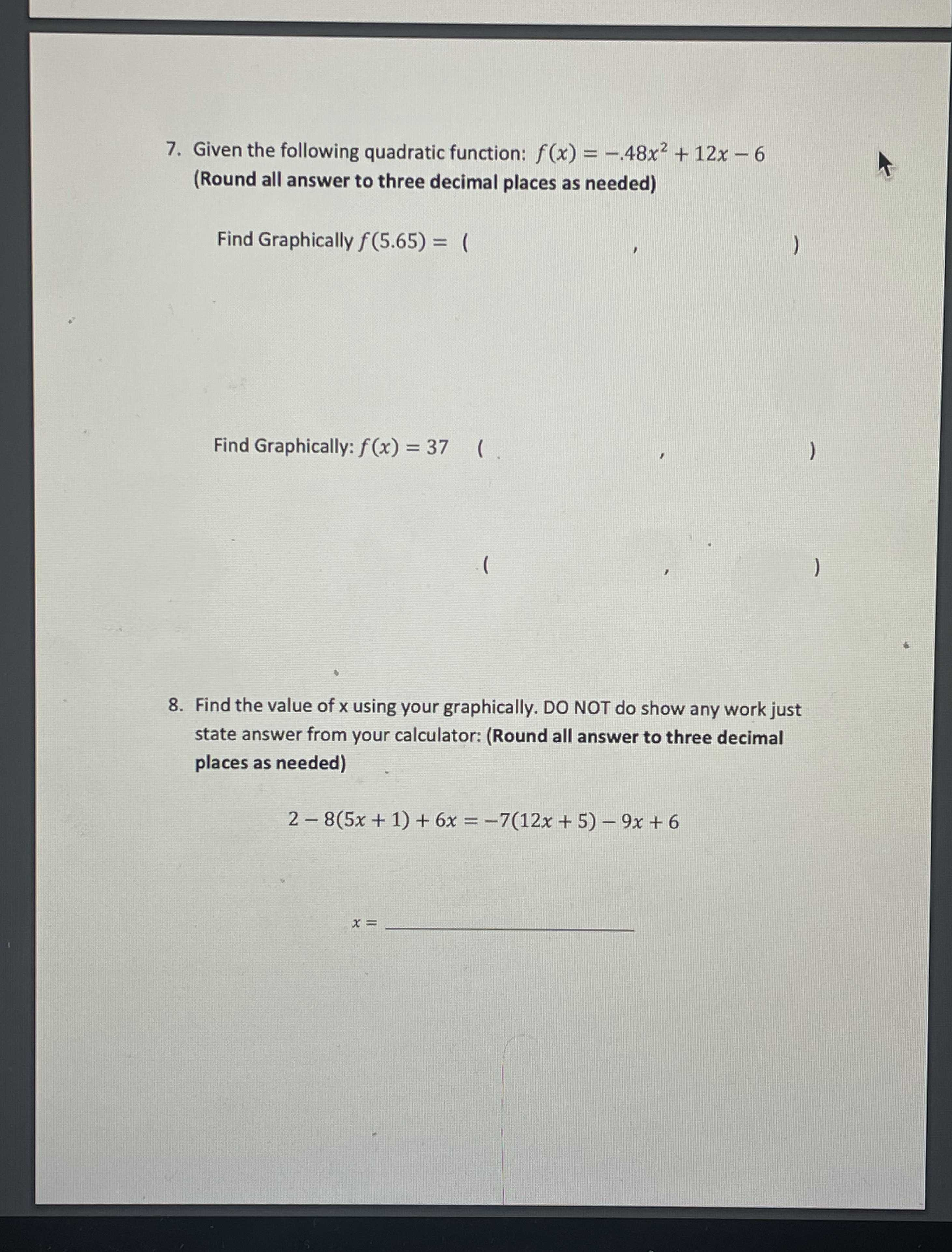### ¿Todavía tienes preguntas de matemáticas?

Pregunte a nuestros tutores expertos
Algebra
Pregunta7. Given the following quadratic function: $$f ( x ) = - .48 x ^ { 2 } + 12 x - 6$$

(Round all answer to three decimal places as needed)

Find Graphically $$f ( 5.65 ) =$$

Find Graphically: $$f ( x ) = 37$$

8. Find the value of x using your graphically. DO NOT do show any work just state answer from your calculator: (Round all answer to three decimal places as needed)

$$2 - 8 ( 5 x + 1 ) + 6 x = - 7 ( 12 x + 5 ) - 9 x + 6$$

$$x =$$